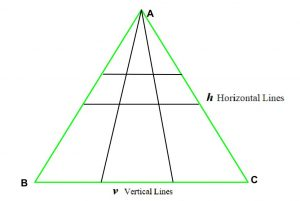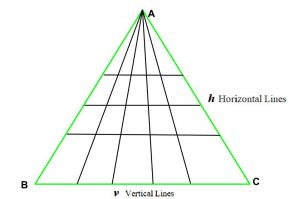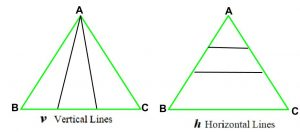# Total number of triangles formed when there are H horizontal and V vertical lines

Given a triangle ABC. H horizontal lines from side AB to AC (as shown in fig.) and V vertical lines from vertex A to side BC are drawn, the task is to find the total no. of triangles formed.

Examples:

Input: H = 2, V = 2
Output: 18As we see in the image above, total triangles formed are 18.

Input: H = 3, V = 4
Output: 60## Recommended: Please try your approach on {IDE} first, before moving on to the solution.

Approach: As we see in the images below, we can derive a general formula for above problem:

1. If there are only h horizontal lines then total triangles are (h + 1).
2. If there are only v vertical lines then total triangles are (v + 1) * (v + 2) / 2..3. So, total triangles are Triangles formed by horizontal lines * Triangles formed by vertical lines i.e. (h + 1) * (( v + 1) * (v + 2) / 2).

Below is the implementation of the above approach:

## C++

 `// C++ implementation of the approach ` `#include ` `using` `namespace` `std; ` `#define LLI long long int ` ` `  `// Function to return total triangles ` `LLI totalTriangles(LLI h, LLI v) ` `{ ` `    ``// Only possible triangle is ` `    ``// the given triangle ` `    ``if` `(h == 0 && v == 0) ` `        ``return` `1; ` ` `  `    ``// If only vertical lines are present ` `    ``if` `(h == 0) ` `        ``return` `((v + 1) * (v + 2) / 2); ` ` `  `    ``// If only horizontal lines are present ` `    ``if` `(v == 0) ` `        ``return` `(h + 1); ` ` `  `    ``// Return total triangles ` `    ``LLI Total = (h + 1) * ((v + 1) * (v + 2) / 2); ` ` `  `    ``return` `Total; ` `} ` ` `  `// Driver code ` `int` `main() ` `{ ` `    ``int` `h = 2, v = 2; ` `    ``cout << totalTriangles(h, v); ` ` `  `    ``return` `0; ` `} `

## Java

 `// Java implementation of the approach ` `class` `GFG { ` ` `  `    ``// Function to return total triangles ` `    ``public` `static` `int` `totalTriangles(``int` `h, ``int` `v) ` `    ``{ ` `        ``// Only possible triangle is ` `        ``// the given triangle ` `        ``if` `(h == ``0` `&& v == ``0``) ` `            ``return` `1``; ` ` `  `        ``// If only vertical lines are present ` `        ``if` `(h == ``0``) ` `            ``return` `((v + ``1``) * (v + ``2``) / ``2``); ` ` `  `        ``// If only horizontal lines are present ` `        ``if` `(v == ``0``) ` `            ``return` `(h + ``1``); ` ` `  `        ``// Return total triangles ` `        ``int` `total = (h + ``1``) * ((v + ``1``) * (v + ``2``) / ``2``); ` ` `  `        ``return` `total; ` `    ``} ` ` `  `    ``// Driver code ` `    ``public` `static` `void` `main(String[] args) ` `    ``{ ` `        ``int` `h = ``2``, v = ``2``; ` `        ``System.out.print(totalTriangles(h, v)); ` `    ``} ` `} `

## C#

 `// C# implementation of the approach  ` `using` `System; ` ` `  `class` `GFG  ` `{  ` ` `  `    ``// Function to return total triangles  ` `    ``public` `static` `int` `totalTriangles(``int` `h, ``int` `v)  ` `    ``{  ` `        ``// Only possible triangle is  ` `        ``// the given triangle  ` `        ``if` `(h == 0 && v == 0)  ` `            ``return` `1;  ` ` `  `        ``// If only vertical lines are present  ` `        ``if` `(h == 0)  ` `            ``return` `((v + 1) * (v + 2) / 2);  ` ` `  `        ``// If only horizontal lines are present  ` `        ``if` `(v == 0)  ` `            ``return` `(h + 1);  ` ` `  `        ``// Return total triangles  ` `        ``int` `total = (h + 1) * ((v + 1) * (v + 2) / 2);  ` ` `  `        ``return` `total;  ` `    ``}  ` ` `  `    ``// Driver code  ` `    ``public` `static` `void` `Main()  ` `    ``{  ` `        ``int` `h = 2, v = 2;  ` `        ``Console.Write(totalTriangles(h, v));  ` `    ``}  ` `}  ` ` `  `// This code is contributed by Ryuga `

## Python3

 `# Python3 implementation of the approach ` ` `  `# Function to return total triangles ` `def` `totalTriangles(h, v): ` `     `  `    ``# Only possible triangle is  ` `    ``# the given triangle ` `    ``if` `(h ``=``=` `0` `and` `v ``=``=` `0``): ` `        ``return` `1` ` `  `    ``# If only vertical lines are present ` `    ``if` `(h ``=``=` `0``): ` `        ``return` `((v ``+` `1``) ``*` `(v ``+` `2``) ``/` `2``) ` ` `  `    ``# If only horizontal lines are present ` `    ``if` `(v ``=``=` `0``): ` `        ``return` `(h ``+` `1``) ` ` `  `    ``# Return total triangles ` `    ``total ``=` `(h ``+` `1``) ``*` `((v ``+` `1``) ``*` `(v ``+` `2``) ``/` `2``) ` ` `  `    ``return` `total ` ` `  `# Driver code ` `h ``=` `2` `v ``=` `2` `print``(``int``(totalTriangles(h, v))) `

## PHP

 ` `

Output:

```18
```

Time Complexity: O(1)
Auxiliary Space: O(1)

My Personal Notes arrow_drop_upCheck out this Author's contributed articles.

If you like GeeksforGeeks and would like to contribute, you can also write an article using contribute.geeksforgeeks.org or mail your article to contribute@geeksforgeeks.org. See your article appearing on the GeeksforGeeks main page and help other Geeks.

Please Improve this article if you find anything incorrect by clicking on the "Improve Article" button below.

Improved By : AnkitRai01, andrew1234